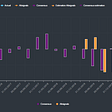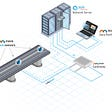# Housing Price Regression

1. Import data, handle missing values / initial data cleaning
2. Explore data by looking at relationships with predictor
3. Transform and preprocess to improve results
`"""Function to perform a forward-backward feature selectionbased on p-value from statsmodels.api.OLSParameters:    X: pandas.Dataframe with candidate features    y: list-like with the target    initial_list: list of features to start with (column names of X)    threshold_in: include a feature if its p-val < threshold_in    threshold_out: exclude a feature if its p-val > threshold_out    verbose: whether to print the sequence of inclusions and   exclusionsReturns: list of selected featuresNote: always set threshold_in < threshold_out to avoid infinite looping"""def stepwise_selection(X, y,                        initial_list=[],                        threshold_in=0.01,                        threshold_out = 0.05,                        verbose=True):        included = list(initial_list)    while True:        changed=False                # forward step        excluded = list(set(X.columns)-set(included))        new_pval = pd.Series(index=excluded, dtype='float64')        for new_column in excluded:            model = sm.OLS(y, sm.add_constant(pd.DataFrame(X[included+[new_column]]))).fit()            new_pval[new_column] = model.pvalues[new_column]        best_pval = new_pval.min()        if best_pval < threshold_in:            best_feature = new_pval.idxmin()            included.append(best_feature)            changed=True            if verbose:                print('Add  {:30} with p-value {:.6}'.format(best_feature, best_pval))# backward step        model = sm.OLS(y, sm.add_constant(pd.DataFrame(X[included]))).fit()        # use all coefs except intercept        pvalues = model.pvalues.iloc[1:]        worst_pval = pvalues.max() # null if pvalues is empty        if worst_pval > threshold_out:            changed=True            worst_feature = pvalues.idxmax()            included.remove(worst_feature)            if verbose:                print('Drop {:30} with p-value {:.6}'.format(worst_feature, worst_pval))        if not changed:            break    return included`
`"""Function to identify multi-collinearity based on a threshold value.Parameters:    data_pred: predictor variables    percent_correlated: threshold percentage to determine the level of correlation                         to identifyReturns: Dataframe of correlated pairs, with duplicates removed"""def multi_collinearity(data_pred, percent_correlated=0.75):    df = data_pred.corr().abs().stack().reset_index().sort_values(0, ascending=False)        # zip the variable name columns (named level_0 and level_1 by default) in a new column named "pairs"    df['pairs'] = list(zip(df.level_0, df.level_1))        # set index to pairs    df.set_index(['pairs'], inplace=True)        # drop level columns    df.drop(columns=['level_1', 'level_0'], inplace=True)        # rename correlation column as cc rather than 0    df.columns = ['cc']        # drop duplicates    df.drop_duplicates(inplace=True)        return df[(df.cc > percent_correlated) & (df.cc < 1)]`
`# import raw datasetraw_data = pd.read_csv('kc_house_data.csv')# examine the raw datasetraw_data.info()`
`clean_data = raw_data.drop('id', axis=1)clean_data['waterfront'] = clean_data['waterfront'].fillna(value=0.)clean_data['yr_renovated'] = clean_data['yr_renovated'].fillna(value=0.)`
`clean_data.duplicated().sum()`
`#convert column to datetimeclean_data['date'] = pd.to_datetime(clean_data['date'],                                     infer_datetime_format=True)# create indidivual columns for year, month, dayclean_data['year_sold'] = clean_data['date'].map(lambda x: x.year)clean_data['month_sold'] = clean_data['date'].map(lambda x: x.month)clean_data['day_sold'] = clean_data['date'].map(lambda x: x.day)# drop date columnclean_data = clean_data.drop('date', axis=1)`
`clean_data.hist(figsize=(25, 25), bins='auto', color='purple');`
`"""Function to plot y vs. a chosen set of x variables: includes regression lineParameters:    data: name of dataframe variables are coming from    target: string of target variable    predictors: dataframe of predictors    nrows: number of rows of subplots    ncols: number of cols of subplots    figsize: size of the figure    type: allows chooser to select between regression or distplot"""def plot_viz(data, target, predictors, nrows=1, ncols=1, figsize=(10, 4), type='reg'):    fig, axes = plt.subplots(nrows=nrows, ncols=ncols, figsize=figsize)        if type == 'reg':        for ax, feature in zip(axes.flatten(), predictors):            sns.regplot(x=feature, y=target, data=data, ax=ax, color='purple', scatter_kws={'alpha':0.2});        plt.tight_layout()`
`# evaluate relationships with pricepredictors = clean_data.drop('price', axis=1)plot_viz(data=clean_data,         target='price',         predictors=predictors,         nrows=5,         ncols=2,         figsize=(15, 15))`
`sns.distplot(clean_data['price']/1000, color='purple')plt.tight_layout()`
`# boxcox transformationclean_data['price_boxcox'], fitted_lamba_0 = stats.boxcox(x=clean_data['price'])print(f'Fitted lambda value: {fitted_lamba_0}')# plot price_boxcoxsns.distplot(clean_data['price_boxcox'], color='purple')`
`# drop price for nowpreprocessed = clean_data.drop('price', axis=1)`
`# plot all continuos against log_pricevariables = ['sqft_living', 'sqft_lot', 'sqft_above', 'sqft_basement']variables_2 = ['yr_built', 'yr_renovated', 'lat', 'long', 'sqft_living15', 'sqft_lot15', 'zipcode']plot_viz(data=preprocessed,         target='log_price',         predictors=variables,         nrows=2,         ncols=2,         figsize=(10, 10),         type='reg')plot_viz(data=preprocessed,         target='log_price',         predictors=variables_2,         nrows=3,         ncols=3,         figsize=(10, 10),         type='reg')`
`# drop clear non-linear columnspreprocessed = preprocessed.drop(['sqft_lot',                                   'yr_built',                                   'zipcode',                                   'lat',                                   'long',                                   'sqft_lot15'], axis=1)# identify and remove any multi-collinearitydata_pred = preprocessed.drop(['log_price', 'price_boxcox'], axis=1)correlated_predictors = multi_collinearity(data_pred, 0.75)correlated_predictors`
`# drop sqft_living, year_sold, sqft_abovepreprocessed = preprocessed.drop(['sqft_living', 'year_sold', 'sqft_above'], axis=1)`
`# create renovated columnpreprocessed['renovated'] = preprocessed['yr_renovated'].apply(lambda x: 1 if x > 0 else 0)# drop yr_renovated columnpreprocessed.drop('yr_renovated', axis=1, inplace=True)`
`# cont. columnsnumerical = ['sqft_basement', 'sqft_living15']numerical_vars = preprocessed_2[numerical]# plot numerical columns against price and see if transformations improve resultsplot_viz(data=preprocessed_2,         target='price_boxcox',         predictors=numerical_vars,         nrows=1,         ncols=2,         type='reg')`
`# plot distributionsfig, axes = plt.subplots(nrows=1, ncols=2, figsize=(10, 4))for ax, feature in zip(axes.flatten(), numerical_vars):    sns.distplot(preprocessed_2[feature], ax=ax, color='purple');plt.tight_layout()`
`preprocessed_2['basement'] = preprocessed_2['sqft_basement'].apply(lambda x: 1 if x > 0 else 0)ohe_list = []ohe_list.append('basement')# drop sqft_basementpreprocessed_2.drop('sqft_basement', axis=1, inplace=True)`
`# transform sqft_living_15 using boxcox transformationpreprocessed_2['t_sqft_liv_15'], fitted_lambda = stats.boxcox(preprocessed_2['sqft_living15'])# drop sqft_living15preprocessed_2.drop('sqft_living15', axis=1, inplace=True)`
`cat_cols = ['bedrooms', 'bathrooms', 'floors', 'waterfront', 'view', 'condition',             'grade', 'month_sold', 'day_sold', 'renovated']cat_predictors = preprocessed[cat_cols]fig, axes = plt.subplots(nrows=5, ncols=2, figsize=(15, 15))for ax, feature in zip(axes.flatten(), cat_predictors):    sns.boxplot(x=feature, y='price_boxcox', data=preprocessed, ax=ax, color='darkred');plt.tight_layout()`
`# identify ordinalordinal_cat_cols = ['bedrooms', 'bathrooms', 'grade']ordinal_predictors = preprocessed_2[ordinal_cat_cols]`
`# replotplot_viz(data=preprocessed_2,         target='price_boxcox',         predictors=ordinal_predictors,         nrows=1,         ncols=3,         figsize=(15, 5),         type='reg')`
`# create dummy variablesbasement_dummies = pd.get_dummies(preprocessed_2['basement'], prefix='basement', drop_first=True)floors_dummies = pd.get_dummies(preprocessed_2['floors_10'], prefix='floors', drop_first=True)water_dummies = pd.get_dummies(preprocessed_2['waterfront'], prefix='water', drop_first=True)view_dummies = pd.get_dummies(preprocessed_2['view'], prefix='view', drop_first=True)condition_dummies = pd.get_dummies(preprocessed_2['condition'], prefix='condition', drop_first=True)month_dummies = pd.get_dummies(preprocessed_2['month_sold'], prefix='month', drop_first=True)day_dummies = pd.get_dummies(preprocessed_2['day_sold'], prefix='day', drop_first=True)reno_dummies = pd.get_dummies(preprocessed_2['renovated'], prefix='reno', drop_first=True)`
`# drop columnsdata_fin_2 = preprocessed_2.drop(ohe_list, axis=1)# concat dummy variables with remaining columnsdata_fin_2 = pd.concat([data_fin_2,                        basement_dummies,                        floors_dummies,                        water_dummies,                        view_dummies,                        condition_dummies,                        month_dummies,                        day_dummies,                        reno_dummies], axis=1)# run stepwise selection to select featuresy = data_fin_2['price_boxcox']X = data_fin_2.drop('price_boxcox', axis=1)result = stepwise_selection(X, y, verbose=True)`
`# run regression with these featuresX = data_fin_2[result]y = data_fin_2['price_boxcox']predictors = sm.add_constant(X)model = sm.OLS(y, predictors).fit()model.summary()`
`# evaluate residuals to check assumptionsdataset = pd.concat([y, X], axis=1)results = []y = 'price_boxcox'for idx, column in enumerate(dataset.columns):    print (f"KC Housing DataSet - Regression Analysis and Diagnostics for {y}~{column}")    print ("-------------------------------------------------------------------------------------")f = f'{y}~{column}'    model = smf.ols(formula=f, data=dataset).fit()        fig, axes = plt.subplots(figsize=(15,12))    fig = sm.graphics.plot_regress_exog(model, column, fig=fig)    fig = sm.graphics.qqplot(model.resid, dist=stats.norm, line='45', fit=True)    fig.tight_layout()    plt.show()        results.append([column, model.rsquared, model.params, model.params, model.pvalues, sms.jarque_bera(model.resid)])`
`# store results in dataframe to make future comparison easymodel_4_results = pd.DataFrame(results, columns=['ind_var',                                                   'r_squared',                                                   'intercept',                                                   'slope',                                                   'p-value',                                                   'normality (JB)'])`
`X = X.drop(['floors_30',            'floors_25',            'view_4',            'view_2',            'view_3',            'view_1',            'water_1',             'condition_2',             'bathrooms'], axis=1)# run regression with these features removedy = data_fin_2['price_boxcox']predictors = sm.add_constant(X)model = sm.OLS(y, predictors).fit()model.summary()`
`# cross validation to make sure we are not overfittingregression = LinearRegression()y = dataset['price_boxcox']crossvalidation = KFold(n_splits=4, shuffle=True, random_state=1)score = np.mean(cross_val_score(regression, predictors, y, scoring='r2', cv=crossvalidation))score# now calc MSEfrom sklearn.metrics import mean_squared_error, make_scorerfrom sklearn.model_selection import cross_val_scoremse = make_scorer(mean_squared_error)cv_results = cross_val_score(regression, X, predictors, cv=5, scoring=mse)mse_score = cv_results.mean()`

--

--

--

## More from Kai Graham

Love podcasts or audiobooks? Learn on the go with our new app.

## Announcing AltSignals.AI [Beta]## Structure Health Monitoring## 4 Factors of a Strong Data Platform## Bayesian Linear Regression Full Derivation## Starbucks Capstone Project## My Journey to Data Science## Analysis of Nigeria Vice-Presidential Debate using Twitter Data## Top Social Science Research Topics in 2020## Logistic Regression — Everything you should know!!## Implementation of Linear Regression## Is multicollinearity always a problem?